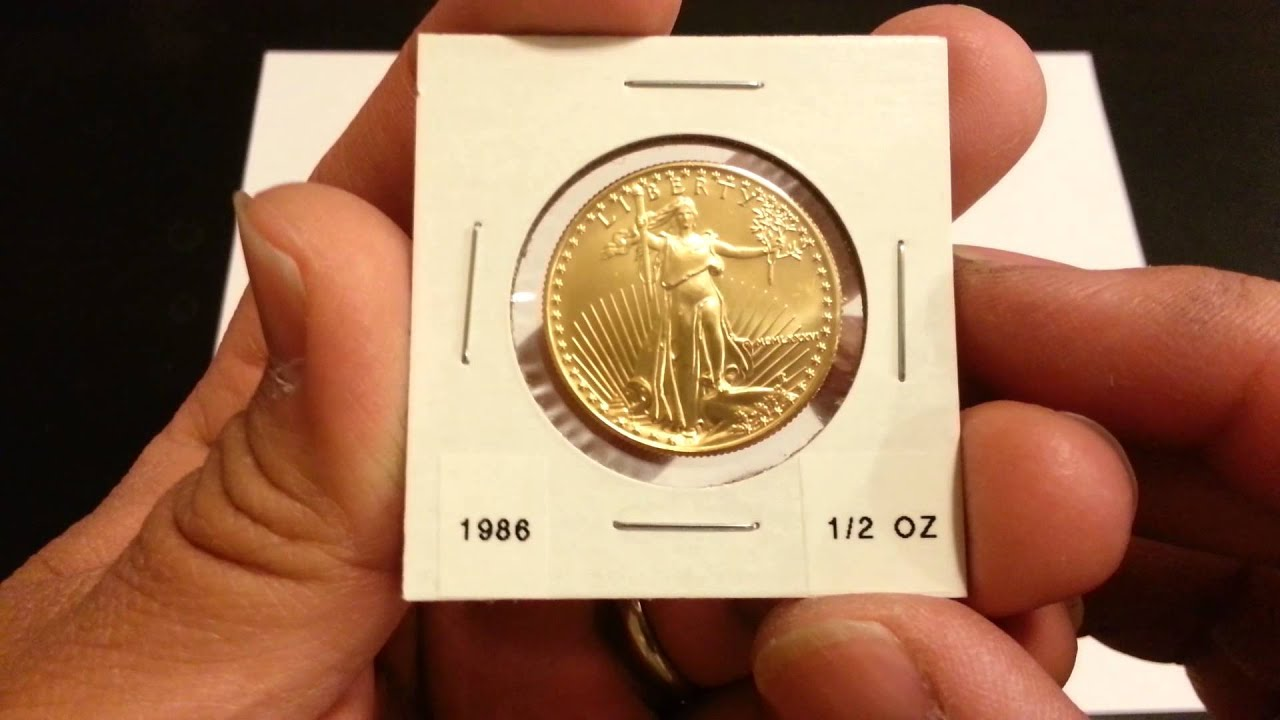## April 21, 2022

### Trade VI: Simplifying to Complexity

I've been working on my algorithms to generate trade networks and manufactured goods. Although probably several years out of date now, Tao's Trade System remains required reading for anyone wanting to do something similar.

This iteration was a much-needed rework of the way a lot of the prices were generated. I had gone through Alexis' work linked above and simplified it to my needs. He is using references based in the real world, whereas I am trying to generate from scratch.

I found that of all the equations, the final price of an object simplified to just a few variables. The first is the local value of a unit (oz) of gold (in cp, all prices are expressed in cp and can then be abstracted back up to sp or gp as needed). I'll call this $g$. To find this number, we need the local availability $g_\ell$ and the total availability $g_t$. $g_t$ is the total number of references reachable from the point in question. This is easy to do with network algorithms. Local availability is a weighted distance calculation over all locations $i$ reachable from $\ell$: $g_\ell = \sum_i \frac{g_i}{\textrm{dist}\left(\ell, i\right) + 1}$ By this calculation, if $i$ has 2 gold references, but is 4 days away, it contributes $\frac{2}{4+1} = 0.4$ references to $g_\ell$.

Right now, I am treating each hex as a node in the graph, but if there is no defined settlement there, all its resources are given to the closest city hex for pricing purposes.

We need a rarity factor $r$ that scales with the size of the network. As the network grows larger, a smaller $r$ is needed to balance things out. I'm trying this out for now: $r = -\frac{1}{n}$ where $n$ is the number of nodes in the network.

The last two constants are the number of gold pieces per oz ($p = \frac{1\textrm{ gp}}{0.48\textrm{ oz}}$) and ratio of cp to gp ($c = \frac{100}{1}$). These are easy to change. A half-ounce gold piece is somewhat hefty; many coins in history would have been much smaller amounts. Gold is valuable enough that a small bit is worth a lot, and hence a great deal of value can be expressed in quite tiny coins. I like the idea of a gp being a weightier coin. I also try to base the sizes of my coins on current analogs that I can actually show to my players.A thousand of these is no joke

So the final equation comes together: $g = \left(r \cdot \frac{g_t}{g_\ell} + 1\right) \cdot p \cdot c$

For an individual resource $q$, the equation is similar. First, we have to define the base cost $b$ of a unit of $q$. I found that this was the most important factor; it essentially represents the ideal economy where everything is in perfect supply. Whether a board-foot of wood is defined as 1 cp or 18 cp will have approximately a 18x effect on the final price of wood no matter where you are in the world. This becomes the key object of research when sketching out the system. It is then an easy matter to determine how many units of $q$ are equivalent to one reference of gold (assuming a gold ref = 1500 oz): $\mathit{ref}_q = \frac{b}{\mathit{ref}_g = 1500\textrm{ oz}}$

We obtain the rarity $r_q$ in a similar way as above with gold, using the distance weighted availability. The final price (in cp) of an item at location $\ell$ is then: $\_q = \frac{g}{\mathit{ref}_q} \cdot \left(r \cdot \frac{q_t}{q_\ell} + 1\right) \mathit{ref}_g$

There are some other ways to view this equation. It can simplify again to: $\_q = \frac{g}{b} \cdot \left(r \cdot \frac{q_t}{q_\ell} + 1\right)$

The next step is to determine the availability of labor references. I haven't quite decided how to assign these so I'll save that for a future post. We obtain the available references by once again iterating on the network: $L_\ell = \sum_i \frac{L_i}{\textrm{dist}\left(\ell, i\right) + 1}$

The cost of a material $m$ at a given stage (eg, hematite $\to$ iron ore) is then the cost of the raw materials (the unit cost $\$_m$times the number of units$n_m\$) plus the labor cost, which is raw material cost divided by the labor references: $\_m = \_q \cdot n_m + \frac{\_q \cdot n_m}{\mathit{ref}_L}$

This step is repeated for each stage of the process, which can be quite complex. I developed a JSON schema to represent each manufactured material. To raise an auroch from a calf to weaned, you require the following.

{
"item": "auroch (weaned)",
"unit": "hd",
"stage": 1,
"tech": 7,
"weight": 200,
"recipe": {
"materials": {
"auroch (calf)": 1,
"min": [
{
"maize": 483,
"oats": 483,
"barley": 483,
"cassava": 483,
"rice": 483,
"wheat": 483
}
]
},
"labor": "herdsman"
}
}


Our raw materials are 1 auroch calf + whichever is cheaper between 483 lbs of feed, plus the labor of a herdsman. As long as every manufactured item "downstream" exists, this "item" will be available for purchase.

## April 8, 2022

### Resources XXIII: Crops and Climates

I've recently completed a little side project that will help resource placement significantly.

I cross-referenced the crop yields from EarthStat with Koppen climate data to get the prevalence for 175 different categories. The data isn't perfect - it doesn't take politics or demographics into account, for example - but I think it will be useful either in automation (as I plan to use it) or in beginning to think about where crops should be placed on a world map.

More details and the data itself can be found here in its own git repo.